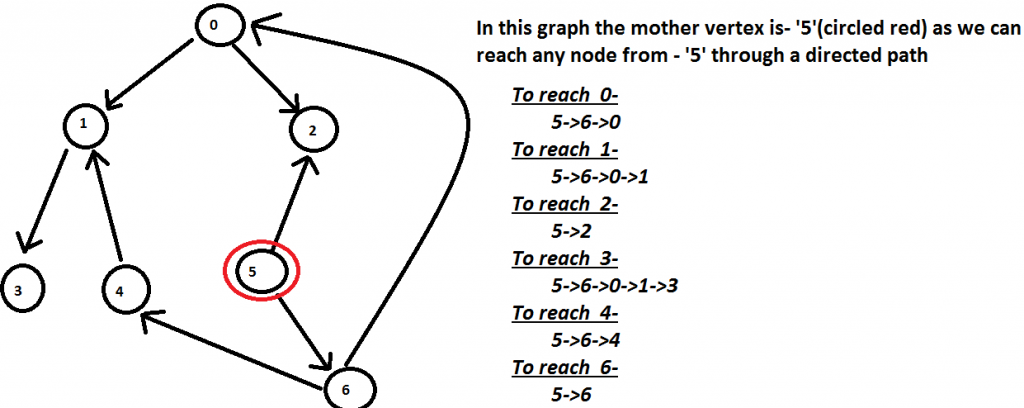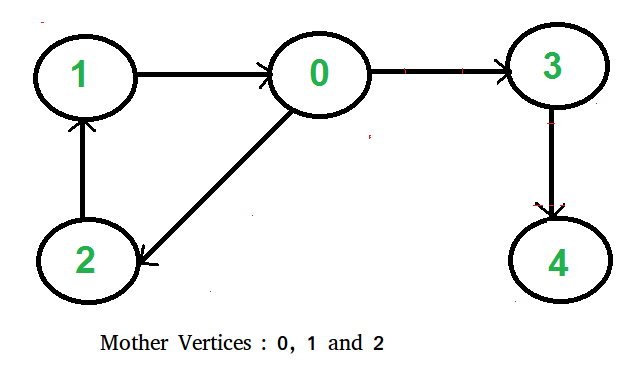GeeksforGeeks App
Open AppBrowser
Continue

# Find a Mother Vertex in a Graph

Write a function to find a mother vertex in the graph.

## What is a Mother Vertex?

A mother vertex in a graph G = (V, E) is a vertex v such that all other vertices in G can be reached by a path from v

Example:

Input: Below GraphOutput: 5

Note: There can be more than one mother vertices in a graph. We need to output anyone of them.
For example, in the below graph, vertices 0, 1, and 2 are mother verticesRecommended Practice

Naive Approach: To solve the problem follow the below idea:

A trivial approach will be to perform a DFS/BFS on all the vertices and find whether we can reach all the vertices from that vertex.

Time Complexity: O(V * (E+V))
Auxiliary Space: O(1) It will be O(V) if the stack space for DFS is considered or if we use a BFS.

## Find a Mother Vertex in a Graph using Kosaraju’s algorithm for strongly connected component:

To solve the problem follow the below idea:

The idea is based on Kosaraju’s Strongly Connected Component Algorithm. In a graph of strongly connected components, mother vertices are always vertices of the source components in the component graph. The idea is based on the below fact:

If there exists a mother vertex (or vertices), then one of the mother vertices is the last finished vertex in DFS. (Or a mother vertex has the maximum finish time in DFS traversal). A vertex is said to be finished in DFS if a recursive call for its DFS is over, i.e., all descendants of the vertex have been visited.

## How does the above idea work?

Let the last finished vertex be v. Basically, we need to prove that there cannot be an edge from another vertex u to v if u is not another mother vertex (Or there cannot exist a non-mother vertex u such that u-→v is an edge). There can be two possibilities.

• A recursive DFS call is made for u before v. If an edge u-→v exists, then v must have finished before u because v is reachable through u and a vertex finishes after all its descendants.
• A recursive DFS call is made for v before u. In this case also, if an edge u-→v exists, then either v must finish before u (which contradicts our assumption that v is finished at the end) OR u should be reachable from v (which means u is another mother vertex).

Follow the given steps to solve the problem:

• Do DFS traversal of the given graph. While doing traversal keep track of the last finished vertex ‘v’. This step takes O(V+E) time.
• If there exists a mother vertex (or vertices), then v must be one (or one of them). Check if v is a mother vertex by doing DFS/BFS from v. This step also takes O(V+E) time.

Below is the implementation of the above approach.

## C++

 `// C++ program to find a mother vertex in O(V+E) time``#include ``using` `namespace` `std;` `class` `Graph {``    ``int` `V; ``// No. of vertices``    ``list<``int``>* adj; ``// adjacency lists` `    ``// A recursive function to print DFS starting from v``    ``void` `DFSUtil(``int` `v, vector<``bool``>& visited);` `public``:``    ``Graph(``int` `V);``    ``void` `addEdge(``int` `v, ``int` `w);``    ``int` `findMother();``};` `Graph::Graph(``int` `V)``{``    ``this``->V = V;``    ``adj = ``new` `list<``int``>[V];``}` `// A recursive function to print DFS starting from v``void` `Graph::DFSUtil(``int` `v, vector<``bool``>& visited)``{``    ``// Mark the current node as visited and print it``    ``visited[v] = ``true``;` `    ``// Recur for all the vertices adjacent to this vertex``    ``list<``int``>::iterator i;``    ``for` `(i = adj[v].begin(); i != adj[v].end(); ++i)``        ``if` `(!visited[*i])``            ``DFSUtil(*i, visited);``}` `void` `Graph::addEdge(``int` `v, ``int` `w)``{``    ``adj[v].push_back(w); ``// Add w to v’s list.``}` `// Returns a mother vertex if exists. Otherwise returns -1``int` `Graph::findMother()``{``    ``// visited[] is used for DFS. Initially all are``    ``// initialized as not visited``    ``vector<``bool``> visited(V, ``false``);` `    ``// To store last finished vertex (or mother vertex)``    ``int` `v = 0;` `    ``// Do a DFS traversal and find the last finished``    ``// vertex``    ``for` `(``int` `i = 0; i < V; i++) {``        ``if` `(visited[i] == ``false``) {``            ``DFSUtil(i, visited);``            ``v = i;``        ``}``    ``}` `    ``// If there exist mother vertex (or vertices) in given``    ``// graph, then v must be one (or one of them)` `    ``// Now check if v is actually a mother vertex (or graph``    ``// has a mother vertex).  We basically check if every``    ``// vertex is reachable from v or not.` `    ``// Reset all values in visited[] as false and do``    ``// DFS beginning from v to check if all vertices are``    ``// reachable from it or not.``    ``fill(visited.begin(), visited.end(), ``false``);``    ``DFSUtil(v, visited);``    ``for` `(``int` `i = 0; i < V; i++)``        ``if` `(visited[i] == ``false``)``            ``return` `-1;` `    ``return` `v;``}` `// Driver code``int` `main()``{``    ``// Create a graph given in the above diagram``    ``Graph g(7);``    ``g.addEdge(0, 1);``    ``g.addEdge(0, 2);``    ``g.addEdge(1, 3);``    ``g.addEdge(4, 1);``    ``g.addEdge(6, 4);``    ``g.addEdge(5, 6);``    ``g.addEdge(5, 2);``    ``g.addEdge(6, 0);` `      ``// Function call``    ``cout << ``"A mother vertex is "` `<< g.findMother();` `    ``return` `0;``}`

## Java

 `// Java program to find a mother``// vertex in O(V+E) time``import` `java.util.*;` `class` `GFG {` `    ``static` `void` `addEdge(``int` `u, ``int` `v,``                        ``ArrayList > adj)``    ``{``        ``adj.get(u).add(v);``    ``}` `    ``// A recursive function to print DFS starting from v``    ``static` `void` `DFSUtil(ArrayList > g,``                        ``int` `v, ``boolean``[] visited)``    ``{``        ``// Mark the current node as``        ``// visited and print it``        ``visited[v] = ``true``;` `        ``// Recur for all the vertices``        ``// adjacent to this vertex``        ``for` `(``int` `x : g.get(v)) {``            ``if` `(!visited[x]) {``                ``DFSUtil(g, x, visited);``            ``}``        ``}``    ``}` `    ``// Returns a mother vertex if exists.``    ``// Otherwise returns -1``    ``static` `int``    ``motherVertex(ArrayList > g, ``int` `V)``    ``{` `        ``// visited[] is used for DFS. Initially``        ``// all are initialized as not visited``        ``boolean``[] visited = ``new` `boolean``[V];` `        ``// To store last finished vertex``        ``// (or mother vertex)``        ``int` `v = -``1``;` `        ``for` `(``int` `i = ``0``; i < V; i++) {``            ``if` `(!visited[i]) {``                ``DFSUtil(g, i, visited);``                ``v = i;``            ``}``        ``}` `        ``// If there exist mother vertex (or vertices)``        ``// in given graph, then v must be one``        ``// (or one of them)` `        ``// Now check if v is actually a mother``        ``// vertex (or graph has a mother vertex).``        ``// We basically check if every vertex``        ``// is reachable from v or not.` `        ``// Reset all values in visited[] as false``        ``// and do DFS beginning from v to check``        ``// if all vertices are reachable from``        ``// it or not.``        ``boolean``[] check = ``new` `boolean``[V];``        ``DFSUtil(g, v, check);``        ``for` `(``boolean` `val : check) {``            ``if` `(!val) {``                ``return` `-``1``;``            ``}``        ``}``        ``return` `v;``    ``}` `    ``// Driver code``    ``public` `static` `void` `main(String[] args)``    ``{``        ``int` `V = ``7``;``        ``int` `E = ``8``;` `        ``ArrayList > adj``            ``= ``new` `ArrayList >();``        ``for` `(``int` `i = ``0``; i < V; i++) {``            ``adj.add(``new` `ArrayList());``        ``}``        ``addEdge(``0``, ``1``, adj);``        ``addEdge(``0``, ``2``, adj);``        ``addEdge(``1``, ``3``, adj);``        ``addEdge(``4``, ``1``, adj);``        ``addEdge(``6``, ``4``, adj);``        ``addEdge(``5``, ``6``, adj);``        ``addEdge(``5``, ``2``, adj);``        ``addEdge(``6``, ``0``, adj);` `          ``// Function call``        ``System.out.println(``"A mother vertex is "``                           ``+ motherVertex(adj, V));``    ``}``}` `// This code is contributed by Tanay Shah`

## Python3

 `# Python3 program to find a mother vertex in O(V+E) time``from` `collections ``import` `defaultdict` `# This class represents a directed graph using adjacency list``# representation`  `class` `Graph:` `    ``def` `__init__(``self``, vertices):``        ``self``.V ``=` `vertices  ``# No. of vertices``        ``self``.graph ``=` `defaultdict(``list``)  ``# default dictionary` `    ``# A recursive function to print DFS starting from v``    ``def` `DFSUtil(``self``, v, visited):` `        ``# Mark the current node as visited and print it``        ``visited[v] ``=` `True` `        ``# Recur for all the vertices adjacent to this vertex``        ``for` `i ``in` `self``.graph[v]:``            ``if` `visited[i] ``=``=` `False``:``                ``self``.DFSUtil(i, visited)` `    ``# Add w to the list of v``    ``def` `addEdge(``self``, v, w):``        ``self``.graph[v].append(w)` `    ``# Returns a mother vertex if exists. Otherwise returns -1``    ``def` `findMother(``self``):` `        ``# visited[] is used for DFS. Initially all are``        ``# initialized as not visited``        ``visited ``=` `[``False``]``*``(``self``.V)` `        ``# To store last finished vertex (or mother vertex)``        ``v ``=` `0` `        ``# Do a DFS traversal and find the last finished``        ``# vertex``        ``for` `i ``in` `range``(``self``.V):``            ``if` `visited[i] ``=``=` `False``:``                ``self``.DFSUtil(i, visited)``                ``v ``=` `i` `        ``# If there exist mother vertex (or vertices) in given``        ``# graph, then v must be one (or one of them)` `        ``# Now check if v is actually a mother vertex (or graph``        ``# has a mother vertex). We basically check if every vertex``        ``# is reachable from v or not.` `        ``# Reset all values in visited[] as false and do``        ``# DFS beginning from v to check if all vertices are``        ``# reachable from it or not.``        ``visited ``=` `[``False``]``*``(``self``.V)``        ``self``.DFSUtil(v, visited)``        ``if` `any``(i ``=``=` `False` `for` `i ``in` `visited):``            ``return` `-``1``        ``else``:``            ``return` `v`  `# Driver code``if` `__name__ ``=``=` `'__main__'``:``  ``g ``=` `Graph(``7``)``  ``g.addEdge(``0``, ``1``)``  ``g.addEdge(``0``, ``2``)``  ``g.addEdge(``1``, ``3``)``  ``g.addEdge(``4``, ``1``)``  ``g.addEdge(``6``, ``4``)``  ``g.addEdge(``5``, ``6``)``  ``g.addEdge(``5``, ``2``)``  ``g.addEdge(``6``, ``0``)` `  ``# Function call``  ``print``(``"A mother vertex is "` `+` `str``(g.findMother()))` `# This code is contributed by Neelam Yadav`

## C#

 `// C# program to find a mother``// vertex in O(V+E) time``using` `System;``using` `System.Collections.Generic;` `class` `GFG {` `    ``static` `void` `addEdge(``int` `u, ``int` `v, List > adj)``    ``{``        ``adj[u].Add(v);``    ``}` `    ``// A recursive function to print DFS starting from v``    ``static` `void` `DFSUtil(List > g, ``int` `v,``                        ``bool``[] visited)``    ``{``        ``// Mark the current node as``        ``// visited and print it``        ``visited[v] = ``true``;` `        ``// Recur for all the vertices``        ``// adjacent to this vertex``        ``foreach``(``int` `x ``in` `g[v])``        ``{``            ``if` `(!visited[x]) {``                ``DFSUtil(g, x, visited);``            ``}``        ``}``    ``}` `    ``// Returns a mother vertex if exists.``    ``// Otherwise returns -1``    ``static` `int` `motherVertex(List > g, ``int` `V)``    ``{` `        ``// visited[] is used for DFS. Initially``        ``// all are initialized as not visited``        ``bool``[] visited = ``new` `bool``[V];` `        ``// To store last finished vertex``        ``// (or mother vertex)``        ``int` `v = -1;``        ``for` `(``int` `i = 0; i < V; i++) {``            ``if` `(!visited[i]) {``                ``DFSUtil(g, i, visited);``                ``v = i;``            ``}``        ``}` `        ``// If there exist mother vertex (or vertices)``        ``// in given graph, then v must be one``        ``// (or one of them)` `        ``// Now check if v is actually a mother``        ``// vertex (or graph has a mother vertex).``        ``// We basically check if every vertex``        ``// is reachable from v or not.` `        ``// Reset all values in visited[] as false``        ``// and do DFS beginning from v to check``        ``// if all vertices are reachable from``        ``// it or not.``        ``bool``[] check = ``new` `bool``[V];``        ``DFSUtil(g, v, check);``        ``foreach``(``bool` `val ``in` `check)``        ``{``            ``if` `(!val) {``                ``return` `-1;``            ``}``        ``}``        ``return` `v;``    ``}` `    ``// Driver code``    ``public` `static` `void` `Main(String[] args)``    ``{``        ``int` `V = 7;``       ``// int E = 8;``        ``List > adj = ``new` `List >();``        ``for` `(``int` `i = 0; i < V; i++) {``            ``adj.Add(``new` `List<``int``>());``        ``}``        ``addEdge(0, 1, adj);``        ``addEdge(0, 2, adj);``        ``addEdge(1, 3, adj);``        ``addEdge(4, 1, adj);``        ``addEdge(6, 4, adj);``        ``addEdge(5, 6, adj);``        ``addEdge(5, 2, adj);``        ``addEdge(6, 0, adj);` `          ``// Function call``        ``Console.WriteLine(``"A mother vertex is "``                          ``+ motherVertex(adj, V));``    ``}``}` `// This code is contributed by Rajput-Ji`

## Javascript

 ``

Output

`A mother vertex is 5`

Time Complexity: O(V + E)
Auxiliary Space: O(V)